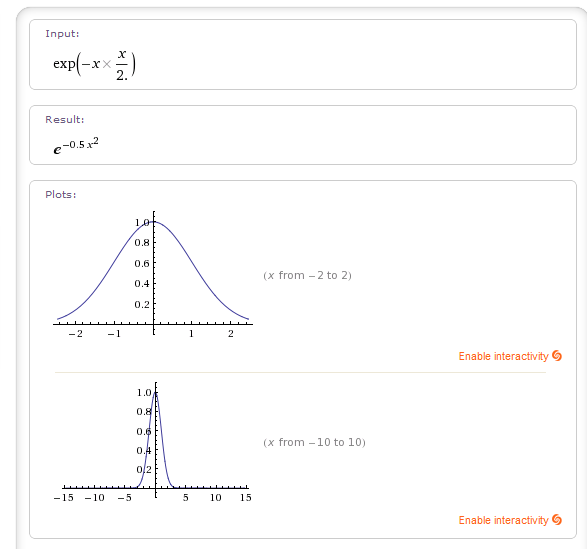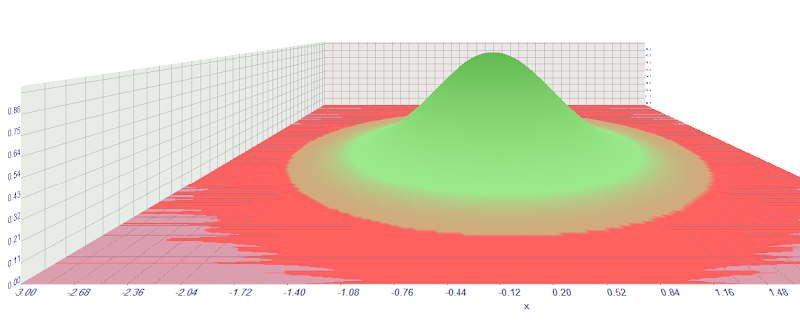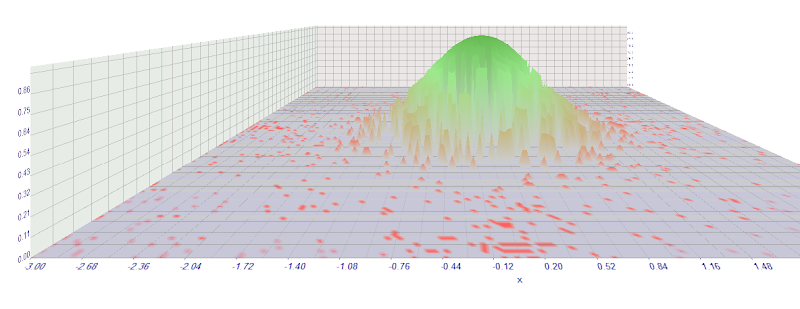# beathespread

• ### Experimental Gaussian kernel function for genetic optimized neural net

Revision created 2713 days ago by JohnLast

• ### Experimental Gaussian kernel function for genetic optimized neural net

Revision created 2713 days ago by JohnLast

• ### Experimental Gaussian kernel function for genetic optimized neural net

Revision created 2722 days ago by JohnLast

• ### Experimental Gaussian kernel function for genetic optimized neural net

Revision created 2722 days ago by JohnLast

• ### Experimental Gaussian kernel function for genetic optimized neural net

Revision created 2722 days ago by JohnLast

• ### Experimental Gaussian kernel function for genetic optimized neural net

Revision created 2722 days ago by JohnLast

## Experimental Gaussian kernel function for genetic optimized neural net: Revision

### Experimental Gaussian kernel function for genetic optimized neural net

Last updated 2722 days ago by JohnLast

I worked recentrly on transfer functions in order to use the native genetic optimizer under Metatrader 4 platform.

We are still at the experimental stage.

1. The sample percetrone code with gaussian transfer function

A percetron code would look like this.

double perceptron()
{
double w1 = MathExp(-x1*x1/2);// This is the Gaussian transfer function
double w2 = MathExp(-x2*x2/2);// Here we can specify the number if the hidden neurones
double w3 = MathExp(-x3*x3/2);
double w4 = MathExp(-x4*x4/2);

double p1 = iCustom(NULL,Symbol(),"PFE",x5,true,5,0,0);// This is the input
double p2 = iCustom(NULL,Symbol(),"PFE",x6,true,5,0,0);
double p3 = iCustom(NULL,Symbol(),"PFE",x7,true,5,0,0);
double p4 = iCustom(NULL,Symbol(),"PFE",x8,true,5,0,0);

return(w1*w2*w3*w4* p1 + w1*w2*w3*w4* p2 + w1*w2*w3*w4*p3 + w1*w2*w3*w4* p4);
}

// From this line depends the whole architecture of the percetrone. We  multiply the input by the value

//coming from the transfer function, in our example it is between 0 and 1.

So this is a sample code. Here we do not devide the output because we are interested if the value is above or below 0.

It is also important that the inputs are normalized and scaled properly. The technical indicator PFE is very appropriate because it is properly scaled and normalized varying frome -1 to +1.

You can see a practical example of the scaling and normalization in the neural indicator code by Jaguar.

Available for registered users.

The use of genetic optimizer limits the number of hidden neurones we can practically use. In the Neuroshell Trader as a commercial software a simmilar application limits itself in the neural net architecture only to two hidden neurones (). And that is considered as a good neural net implementation practice. (Neural indicators for Neuroshell are available as additional addon, you can refer to the help files).

2. The transfer function

As it was previously stated we can choose different transfer functions in this part of the code.

The gaussian transfer function is:

double w1 = MathExp(-x1*x1/2);

x1 means in practice that we are looking for the best avalues of x1 using the genetic optimizer.

the value of x would be from -3 tp +3. In practice I can suggest a step size of 0.01.От 08 ноември 2011

3. Towards a more sophisticated transfer function.

Yesterday I watched a very impressive video about the possibilities of Metatrader 5 for visualisation of the 3 D visualisation space.

So then came into my mind the idea, as we are limited with the genetic optimizer by the number of the hidden neurones we can use (of course you can use many hidden neurones but you will otpimize forever).

So why not to use just one, or two hidden neurones but we will have two parameters in the transfer function, not just one.

So the code will look like this:

double w1 = MathExp(-x1*x1-y1*y1);

Here we optimize two parameters x1 and x2.

On the first shot you can see what you will get if you do not use a genetic optimizer.От 23 септември 2011

Here on the second shot is what you will practically get using a genetic optimizer searching for the best values of x and y.

For the variable x the start os -3, the step is 0.01 and the stop is 3

For the variable y the start os -3, the step is 0.01 and the stop is 3

So the idea is that we just one hidden neurone using a genetic optimization with two variables we could achieve 3 dimensional  hypersphere with just one radial unit.От 23 септември 2011